All spreadsheets are professionally prepared and presented.

These spreadsheets will save you time and hassle, and cover a wide range of financial modeling concepts.

## Pricing European Swaptions

This Excel spreadsheet employs the Black (1976) model to price European interest rate options. All of the calculations are exposed to ensure clarity.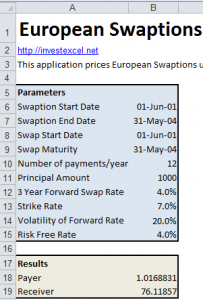## Pricing Foreign Exchange Options

This spreadsheet uses the Garman-Kohlhagen model to calculate the price of a European foreign currency option. Moreover, the spreadsheet also calculates if put-call parity is satisfied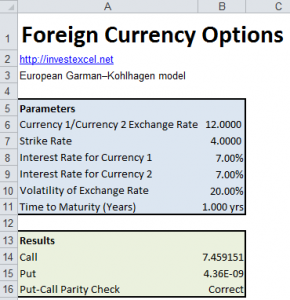## Pricing Caplets and Floorlets

This Excel spreadsheet gives the price of a caplet and floorlet using the Black 76 model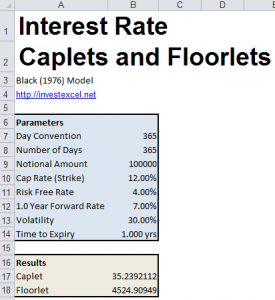## Pricing Lookback Options (Fixed & Floating Strikes)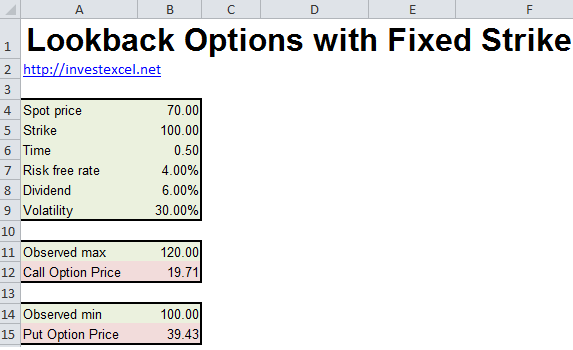## Pricing Asian Options

This Excel spreadsheet gives the price of an Asian Option based on geometric averages (Kemna & Vorst, 1990) and arithmetic averages (Levy, 1991)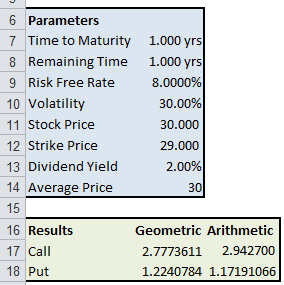## Price an American Option with a Trinomial Tree

This Excel spreadsheet prices an American Option with a Trinomial Tree. An American option is a financial instrument that lets the owner buy (call) or sell (put) a stock at or before an agreed maturity time.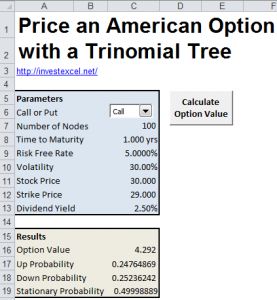## European Options under Jump Diffusion

This Excel spreadsheet implements Merton’s classic model for pricing European options under the influence of jump diffusion.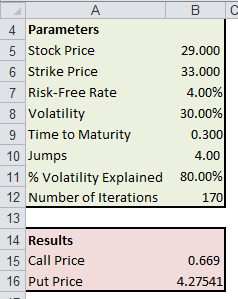## Price an American Option with a Binomial Tree

Price an American option (Call or Put) with no dividends.  This spreadsheet also generates the pricing lattice automatically for you to view.

## Maximize the Omega Ratio of a Portfolio

This Excel spreadsheet finds the investment weights that maximize the Omega Ratio of a portfolio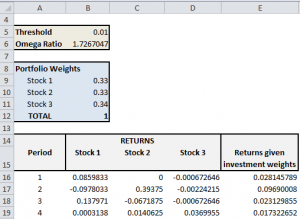## Implied Volatility

This spreadsheet calculates the implied volatility of a European Option priced with Black-Scholes.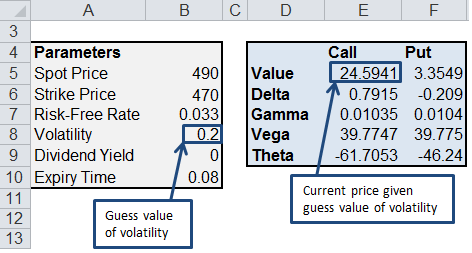## Calculate a Sharpe Optimal Portfolio with Excel

This Excel spreadsheet will calculate the optimum investment weights in a portfolio of three stocks by maximizing the Sharpe Ratio of the portfolio.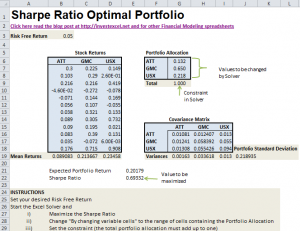## Calculate the Sharpe Ratio with Excel

This spreadsheet provides a worksheet function and VBA code that calculates the Sharpe Ratio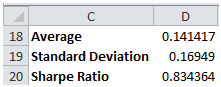## Calculate the Omega Ratio with Excel

The Omega Ratio is a financial benchmark created by Shadwick and Keating in 2002. The Omega Ratio captures all the information in the entire returns distribution, correctly modeling the effect of low-probability, high-impact events (i.e. fat tails).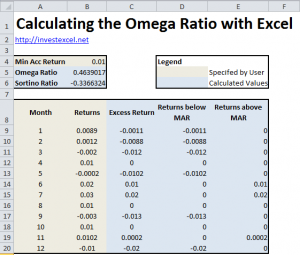## Calculate Modified Value at Risk with Excel

A spreadsheet that models skew and kurtosis in the returns distribution to calculate the Value at Risk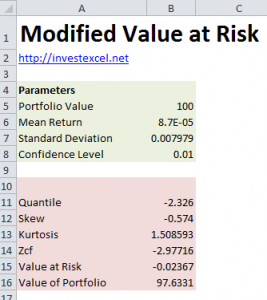## Calculate the Modified Sharpe Ratio with Excel

Modifies the standard Sharpe Ratio to model skew and kurtosis in the returns distribution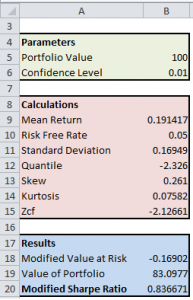## Markowitz Portfolio Optimization with Excel

An easy-to-use spreadsheet that implements Markowitz’s classic mean-variance optimization approach to find the best distribution of stocks in a portfolio.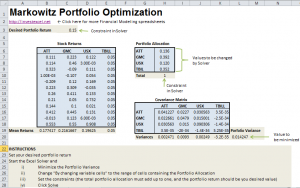## Calculate Kappa with Excel

Kappa is a generalized downside-risk adjusted performance measure.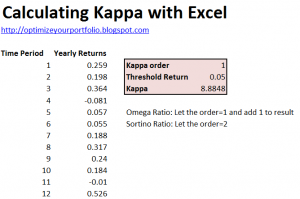## Import Historical Forex Rates in Excel by Connecting to a Web Service

This Excel spreadsheet downloads historical Forex data from the Internet, specifically the daily bid rates.  Just enter two three-letter currency symbols, two dates, and click a button.

The spreadsheet will then connect to a remote website, and download daily bid rates (using some clever VBA).  The Forex data is imported into a sheet called “Data”.

Now you can analyze and plot the forex data using all of Excel’s functionality. As an example, the spreadsheet plots the exchange rate data.  If you wanted, you could easily add Bollinger Bands to the plot.

## Calculate Downside Deviation with Excel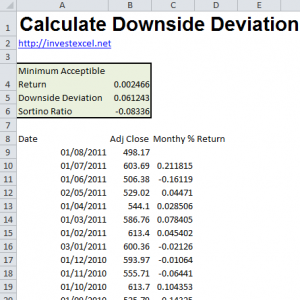## Calculate Jensen’s Alpha with Excel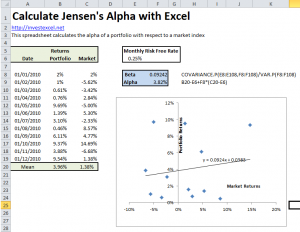## Calculate Stock Beta with Excel## Calculate Value at Risk with Excel

This spreadsheet implements the standard Value at Risk calculation, based on a normal distribution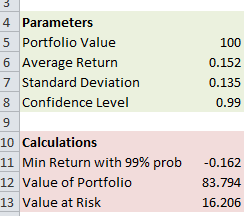## Calculate Value at Risk Based on Monte-Carlo Simulation

This spreadsheet calculates Value at Risk based upon Monte-Carlo simulation.  The VBA code can be viewed, and used in your own spreadsheets.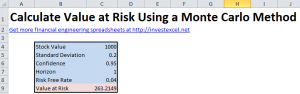Once you click the button, Excel  connects to Yahoo Finance and retrieves daily stock quotes, placing the data into a sheet called “Data”

Currently, the spreadsheet imports the date (in day/month/year format), opening price, daily high, daily low, closing price, trading volume and the adjusted close.  All prices are in US dollars.

## Calculate the Information Ratio with Excel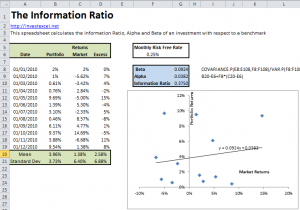## Calculate the Treynor Ratio with Excel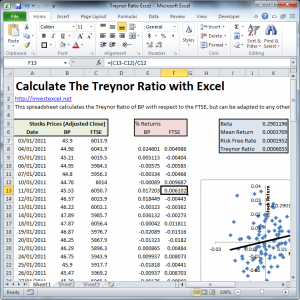## Black-Scholes and Greeks Calculator for Excel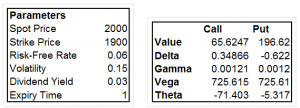### 8 thoughts on “Financial Modeling Spreadsheets”

1. This site has valuable resources and I will visit this site often.

• Hi Samir,
Could you please give me an email address where I can contact with you directly.
Regards,
Istvan.

• Hello Samir,

I was looking for some resource to understand corporate actions on listed/unlisted stocks.

I must add that going through the sheets and the vba, helped me understand the stuff much easier than the amount of time spend to read the theory.

I am not advising against reading theory, but I tend to understand better this way.

You are really doing a great job with this site and resources made available here. All i can say is well done. GOD Bless You.

If it is alright i would love to keep in touch with you, going forward.

Look forward to hearing from you.

Regards

Jacob

2. Great website with invaluable tools.

Suggestion: It would be great if the “Sharpe Ratio Optimal Portfolio” spreadsheet could also graph/plot the efficient frontier curve and data.

I use solver to find the optimal weights of the 20 stocks for the the lowest variance portfolio, and calculate the weights of the stocks from this point to the highest possible return portfolio. How do I find the points in between? For example, the return on my minimum variance portfolio is 1, the return on my max return portfolio is 100. Right now, I would need to run optimizer with minimum variance for the returns of 2,3,4….all the way to 99 to find those points. There got to be a more efficient way to do this for plotting the points and graphing the curves.

Thanks.